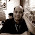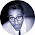## Sunday, June 6, 2010

### Python, Econometrics: Granger Test for Causality at version 0.0.2

Draft June.06.2010 No example yet.

Does variable X causes Y or vice-versa? We say that X granger cause Y if past values of X helps in predicting future values of Y. In other words, in the the model $$Y = \sum \alpha_i X_{t-i} + \sum \beta_i Y_{t-i} + u_t$$, computed estimates of $$\alpha_i$$ are significantly non zero.

In a similar manner, Y granger cause X if past values of Y helps in predicting future values of Y. Thus in the model $$X = \sum \delta_i X_{t-i} + \sum \gamma_i Y_{t-i} + u_t$$, the estimated value of $$\gamma_i$$ are significantly different from zero.

Here is (untested!)Python code for performing a Granger test on whether a variable X causes Y (or X preceeds Y)

"""
"""
File       grangertest.py

author  Dr. Ernesto P. Adorio
UPDEPP, UP CLARK

version 0.0.1  06.06.2010
version 0.0.2  06.09.2010
"""

from matlib import *
import lm
from scipy import stats

def grangertest(Y,X,  maxlag):
"""
Performs a Granger causality test on variables (vectors) Y and X.
The null hypothesis is: Does X causes Y?
Returned value: pvalue, F, df1, df2
"""
# Create linear model involving Y lags only.
n = len(Y)
if n != len(X):
raise ValueError,  "grangertest: incompatible Y,X vectors"
M = [  * maxlag for i in range(n-maxlag)]
for i in range(maxlag,  n):
for j in range(1,  maxlag+1):
M[i-maxlag][j-1] =  Y[i-j]

fit = lm.ols(M, Y[maxlag:])

# Create linear model including X lags.
for i in range(maxlag,  n):
xlagged = [X[i-j] for j in range(1,  maxlag+1)]
M[i-maxlag].extend(xlagged)
fit = lm.ols(M, Y[maxlag:])
df1 = maxlag
df2 = n - 2 * maxlag - 1
pvalue = 1.0 - stats.f.cdf(F,  df1,  df2)
return pvalue, F, df1,  df2,   RSSr,  RSSu

def Test():
years,  chicks ,  eggs = [], [],  []
for line in D:
splitted = line.split()
if len(splitted) == 3:
year,  chick,  egg = splitted
years.append(float(year))
chicks.append(float(chick))
eggs.append(float(egg))

lag = 4
print "Ho: do chicks cause eggs?"
pvalue,  F,  df1,  df2,  RSSr,  RSSu =grangertest(eggs,  chicks,  maxlag = lag)
print "pvalue, F, df1, df2, RSSr, RSSu=",  pvalue ,  F, df1,  df2,  RSSr,  RSSu

print "Ho: do eggs cause chicks?"
pvalue,  F,  df1,  df2,  RSSr,  RSSu =grangertest(chicks, eggs,  maxlag = lag)
print "pvalue, F, df1, df2, RSSr, RSSu=",  pvalue ,  F, df1,  df2,  RSSr,  RSSu

if __name__ == "__main__":
Test()


The test data file may be downloaded from uiuc econometrics group: data eggs.

we are wondering why the data eggs has 77 rows while the example programs in that reference shows only 53 observations! We will go back to this later but for a lag of 4, Here are the results.

python grangertest.py
Ho: do chicks cause eggs?
pvalue, F, df1, df2, RSSr, RSSu= 0.919252836295 0.232357141261 4 68 1401289.91664 1382395.24562
Ho: do eggs cause chicks?
pvalue, F, df1, df2, RSSr, RSSu= 0.00172519529214 4.83191020278 4 68 30442292479.4 23704704139.1

We will be happy if a reader shows us where the discrepancy lies. The published example shows pvalue of

The Null hypothesis is that X does not granger cause Y. If the returned pvalue is less than specified alpha, then the Null hypothesis is rejected, otherwise we fail to reject the hypothesis.

We will provide an example later. Classes will be opening soon and we have to do something else at the moment.

Reference: 696-700 of Gujarati

Wikipedia: Wiki article on Granger Causality

Last WARNING: I still need an alternate published example to check the veracity of this code.

1.2.3.